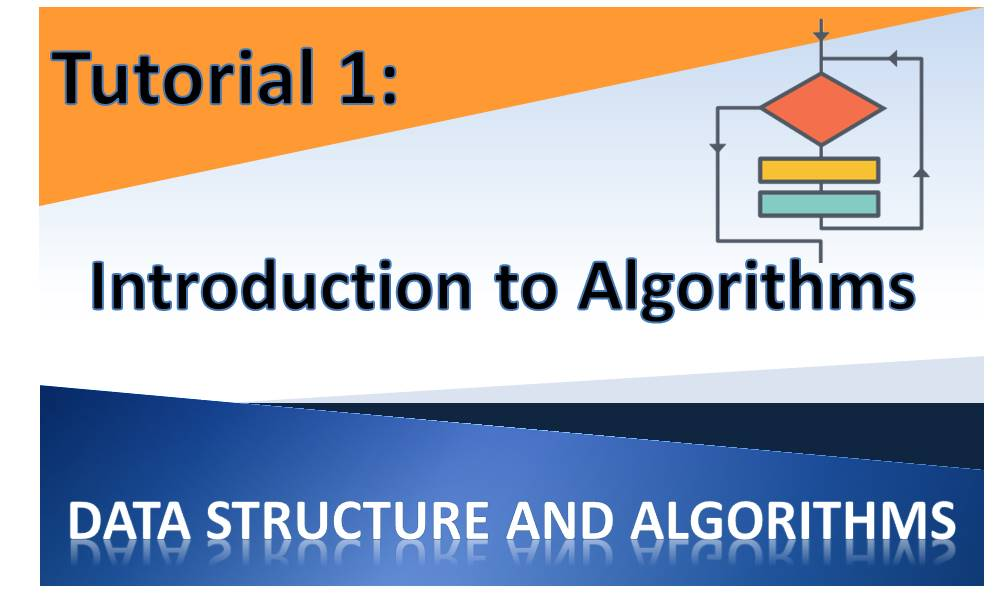# Data Structures and Algorithms Tutorial 1 – Introduction to AlgorithmsThis is a series of tutorial on Data Structures and Algorithms for Computer Science/Engineering students.
After taking this tutorial you would be able to write any algorithm as well as write the program in any programming language.

What we are going to cover in Tutorial 1:

### 1. What is an Algorithm

An Algorithm is defined as “a well-defined computational procedure that takes as input a value or set of values and then produces a value or set of values a its output”.
In other words, an algorithm is a sequence of steps taken to process a given input to produce an output.
We can also describe an algorithm as a procedure for solving computational problems

### 2. Sorting Problem Example

How can we use an algorithm to solve a sorting problem? We can do that in three steps:
Step 1: Determine the input
Step 2: Determine the output
Step 3 Define the relationship

In this case we have:
Input: A sequence of Numbers (a1, a2, … , an)
Output: A reordering of the input sequence (a1, a2, … , an)
Relationship: The reordering is such that a1 is ≤ a2 ≤ … ≤ an

### 3. Factors Determining Choice of Algorithms

An algorithms may be able to solve the given problem. In that case, we say it is correct. But that does not mean it is the algorithm of choice for the problem
The following factors determine the choice of algorithm to use for particular problems:

• The number of items to be sorted
• Any constraints on the value of the items
• The architecture of the computer to be used
• Type of storage device (eg memory, disk, tape etc)

### 4. Applications of Algorithms

Why are algorithms so important in computing and problem solving? This is because it applies to a wide array of areas. Let’s look at some areas:

In DNA Data Storage and Analysis: the human DNA contains 100,000 genes and is made up of 3 billion chemical base pairs.
This this data needs to be stored for hundreds of millions of people such that when DNA tests are carried out on someone, the persons DNA data could be compared to the stored record. To achieve this storage and comparison, complex algorithms are used to develop the required tools.

Internet Traffic: How long does it take to search through your computer for a particular file if you have only the name or just part of the name? Now think of the internet with millions of millions of petabytes of data located in countless number of disks in datacentres around the globe. Sophisticated algorithms make is possible to perform this search in the shortest possible time. So next time you visit Google to do a search and get results in few seconds, you should be appreciate the amount of work we Computer Scientists have put into place!

E-commerce: Complex algorithms are used to manage large volume of transactions online on E-commerce sites. This includes managing millions of credit card information and data transfer between banks and other organizations while maintaining privacy requirements.

Resource Allocation: In the manufacturing process and other industries, there is need for scarce resources to be allocated in the most beneficial manner in order to minimize cost of production and to maximize profit. This is achieved using algorithms behinds the information systems used in managing the processes.

Other Application: Other application areas of algorithms include:

• Finding shortest distance between two locations
• Finding a convex hull of a set of points
• Finding sequences in a large number of dataset etc

### 5. Characteristics of Algorithms

There are two main characteristics of algorithms you need to know:

• Algorithms have more than one possible solutions
• Algorithms have practical applications

### 6. What are Data Structures

A data Structure is an arrangement for  storing and organizing data to enable easy access and operations. By now you should be familiar with a number of data structures. The simple ones includes Arrays, Lists, Queue, Stack and Linked list. Others are Dictionary and Hash tables. We would examine all of this in subsequent posts.

### 7. What is an NP-Complete Problem

NP-Complete problem is a term in algorithms used to refer to a problem  that has no efficient solution. There may be algorithms written but the solution cannot be described as efficient.
This concept is important because if a problem is proven to be an NP-Complete problem, then you need not spend the time trying to develop an efficient algorithm.

### 8. What is Parallelism

This applies to processors such that a number of processors work simultaneously to perform an algorithmic operation. You may be familiar with terms like dual-core, quad-core etc as it applies to CPUs in the modern computer systems.
This concept is important to know because to design an efficient algorithm, we need to have the concept of parallelism in mind.

### 9. Final Notes

I hope that this introductory note on Data Structures and Algorithms is clear enough. I would try to keep this tutorial simple and clear. I would also be interacting as we would be implementing out algorithms in different programming languages and actually running them on the computer. I would give a step-by-step of every algorithm and program we would write.